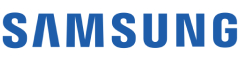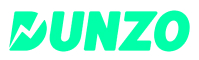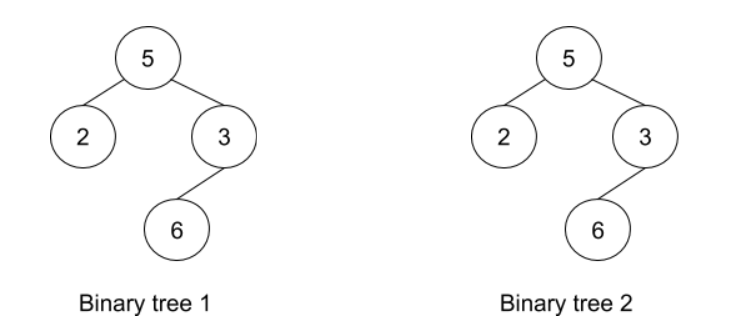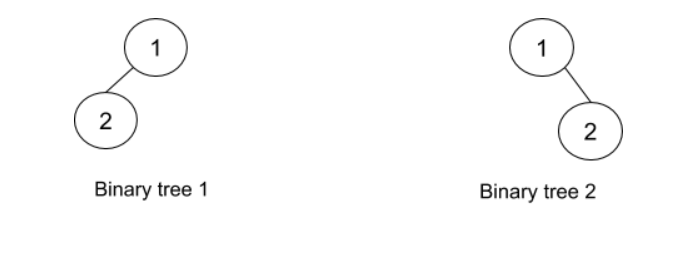New update is available. Click here to update.
Topics

# Check Identical Trees

Moderate0/80
Average time to solve is 20m+15 more companies

## Problem statement

You are given two binary trees with 'n' and 'm' nodes respectively.

You need to return true if the two trees are identical. Otherwise, return false.

Example:
``````For the trees given below:-
````````````The given trees are identical as:-
1. The number of nodes in both trees is the same.
2. The number of edges in both trees is the same.
3. The data for root for both the trees is the same i.e 5.
4. The data of root -> left (root’s left child) for both the trees is the same i.e 2.
5. The data of root -> right (root’s right child) for both the trees is the same i.e 3.
6. The data of root -> right -> left ( left child of root’s right child) for both the trees is the same i.e 6.
7. Nodes with data 2 and 6 are the leaf nodes for both the binary trees.
``````
Detailed explanation ( Input/output format, Notes, Images )
Sample input 1 :
``````5 2 3 -1 6 -1 -1 -1 -1
5 2 3 -1 6 -1 -1 -1 -1
``````
Sample output 1 :
``````True
``````
Explanation for Sample Input 1 :
``````Refer to the example given above in the problem description.
``````
Sample input 2 :
``````1 -1 2 -1 -1
1 2 -1 -1 -1
``````
Sample output 2 :
``````False
``````
Explanation for Sample Input 2 :``````Although the following conditions satisfy:

The number of nodes in both trees is the same.
The number of edges in both trees is the same.
The data for root for both the trees is the same i.e 1.

It’s visible from the pictorial representation that the trees are not identical. Reason being:
In Binary tree 1, root’s right is NULL but it’s not true for Binary tree 2.
In Binary tree 1, root’s left is not NULL but it’s not true for Binary tree 2.
``````
Expected time complexity:
``````The expected time complexity is O(min(n,m)).
``````
Constraints :
``````0 <= 'n' <= 10^6
0 <= 'm' <= 10^6
1 <= Node Data <= 10^9

Time limit: 1 sec
``````Console